Introduction to Hypothesis Testing

We will present a basic hypothesis testing procedure and apply it to an example problem to illustrate some of the concepts.

Key Terms

o       Statistical hypothesis

o       Hypothesis testing

o       Null hypothesis

o       Alternative hypothesis

o       Significance level

o       Test statistic

o       Critical value

Objectives

o       Understand the basic concepts and terminology of hypothesis testing

o       Recognize the steps of a hypothesis testing procedure in an example problem

Characterizing data according to means, standard deviations, regression lines, and so forth may be satisfying in its own right, but we also want to be able to make conclusions about data according to some measure of statistical significance. The ability to decide such matters as whether a die or coin is actually "fair" based on a series of trials. Hypothesis testing allows us to apply our statistical knowledge to this and other such problems.

Elements of Hypothesis Testing

Before discussing the mathematical aspects of hypothesis testing, we must define some terms and develop a systematic process by which we evaluate some aspect of a problem. Let's define a statistical hypothesis as some statement regarding the distribution of a random variable. For some random variable X, this hypothesis might be as simple as "the mean of the distribution of X is a," or it might be a more involved statement, such as "the six-sided die is fair." Given the assumption of some statistical hypothesis, we wish to perform hypothesis testing, which is the process by which we determine whether we should reject or not reject (that is, accept) the hypothesis. (Note that the alternatives in hypothesis testing are generally reject and not reject, rather than accept and reject--this is simply because the hypothesis is assumed to be true at the outset of the investigation. Hypothesis testing then determines if the assumption was not warranted based on the available data--if it is not rejected, then one continues to assume it is true. Other hypotheses may be better, however.)

The statistical hypothesis under test is often called the null hypothesis, with the negation of the null hypothesis being the alternative hypothesis. We will assign the symbol H0 to the null hypothesis and the symbol Ha to the alternative hypothesis. As mentioned, we also want to have some measure of statistical significance--hypothesis testing generally uses the symbol α for the significance level, which is a percentage that is chosen arbitrarily as the measure of what qualifies as a statistically significant deviation of a statistical value from an assumed value. To test our hypothesis, we also require a test statistic that allows us to evaluate the data in light of the hypothesis and an associated critical value that allows us to determine whether or not to reject the hypothesis. For instance, given a random variable X for which we want to test some hypothesis H0 (versus an alternative hypothesis, Ha), we can write the following equation: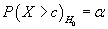This equation simply states that the probability that the random variable X exceeds some critical value c (given the null hypothesis H0) is the significance level α. In other words, our procedure should involve choosing a critical value c for the test statistic so that if the random variable exceeds this value, we conclude that the data shows a statistically significant deviation from the null hypothesis. After formulating our procedure for hypothesis testing, we will go through an example that illustrates these concepts.

Process of Hypothesis Testing

The process of hypothesis testing can be summarized in the following way. All you need to do at this point is get a sense of the logic; you will get a better sense of the process when we present an example in the next section.

1.      Formulate the null hypothesis, H0, and the alternative hypothesis, Ha, for the problem. (This step may require more careful consideration than may be apparent at first glance.)

2.      Select a significance level, α, that defines the probability of a statistically significant deviation. (The values 0.01 and 0.05 are two common choices for α.)

3.      Choose an appropriate test statistic for evaluating the hypothesis.

4.      Calculate the critical value, c, according to the test statistic and the significance level, α.

5.      Evaluate the data for the random variable and determine if it exceeds the critical value, c, for the test statistic. If so, then the null hypothesis should be rejected; if not, then the null hypothesis should not be rejected.

Again, this procedure is best understood by way of an example.

Interested in learning more? Why not take an online Statistics course?

Hypothesis Testing Example

Consider a situation where a statistician is attempting to determine whether a coin is a "fair" coin. (A fair coin is one that, on average, turns up heads 50% of the time and tails 50% of the time.) Obviously, in a given number of trials there is a finite probability that the coin turns up heads more often than tails (or vice versa)--the statistician needs a process to determine whether such variation in a given set of trials is statistically significant. Let's consider this problem using our hypothesis testing procedure.

First, we need to formulate our null hypothesis, H0, and the alternative hypothesis, Ha. Assume the data shows more heads turning up than tails in a trial of a certain number of flips. The analysis will proceed on the assumption that the coin is fair, however; thus,

H0: The coin is not weighted toward heads (the probability of the coin turning up heads is 0.5).

Ha: The coin is is weighted toward heads.

Next, we must select a significance level for the problem. As mentioned previously, 0.01 and 0.05 (1% and 5%, respectively) are common choices--let's choose α = 0.01. We'll discuss what this means after we choose the test statistic. Our choice of a test statistic involves determining a random variable that we can use in the hypothesis testing procedure. The random variable for this analysis can potentially be expressed in various ways, but we will say that the random variable X is defined as follows:

X = Number of heads in x flips

Now, assume we have a data set consisting of the results of 5,000 trials (flips of the coin in question), and X is 2,576. Note that there were not exactly 2,500 heads--we want to find out if this difference of 76 is a statistically significant deviation from the expected value of 2,500. We must now determine a critical value c against which we can compare X = y. Rewriting the probability expression from above for this situation,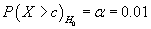This statement, applying our choice of α = 0.01, means that the probability that X exceeds some critical value c is 0.01. To calculate c, we need to know the probability distribution of X. In this case, X must be a discrete distribution, but let's assume that X follows the normal distribution (this is actually a decent approximation) with a mean of 2,500 and a variance of 1,250. Recall from our study of the normal distribution that we can use a table of values to find the critical value, c. Let's rewrite the probability expression from above: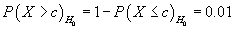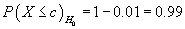We can calculate the Z-score of c using the standard normal distribution and then convert this Z-score to a c value using the following formula.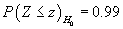z = 2.33

Given the mean (2,500) and variance (1,250) of the distribution,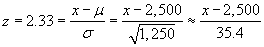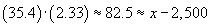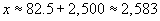Thus, we now have a complete expression for our hypothesis test: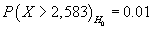What exactly does this expression mean? It simply means that the probability that the number of heads exceeds 2,583 in 5,000 trials is 0.01. We can now compare the value of X, our random variable, to the critical value, c.

X = 2,576 ≤ 2,583 = c

Thus, because X = 2,576 does not exceed the critical value of c = 2,583, we do not reject the null hypothesis, H0, and we continue to proceed on the assumption that the coin is a fair coin. Had the data indicated that X = 2,601, for instance, then we would have been justified, according to our procedure, in rejecting the null hypothesis and instead proceeding on the assumption that the alternative hypothesis is true.

Review of Hypothesis Testing

We have thus outlined and illustrated through example the basic hypothesis testing procedure. We have not, however, provided all the details regarding how to determine the distribution of the test statistic (random variable)-in some cases, this is a complicated problem. Nevertheless, you should at this point be able to apply hypothesis testing to simple problems where some of this information is either provided or is otherwise easily accessible. The practice problems and exercises below help reinforce the concepts that we have studied.

Practice Problem: Assume that the details of the example problem above are the same, except that X = 2,561. For a significance level of 0.05, determine whether the null hypothesis should be rejected.

Solution: All we need to do to solve this problem is follow the pattern provided earlier (and, specifically, in the example problem) and simply replace some of the numbers. For α = 0.05,Then,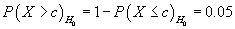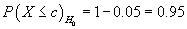For the Z-score in this case,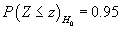z = 1.65

Now, let's calculate the value of c corresponding to this Z-score.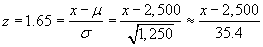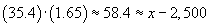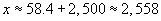Because the value of X = 2,561 exceeds c ≈ 2,558, we are justified in rejecting the null hypothesis and in proceeding on the assumption that the coin is not a fair coin.